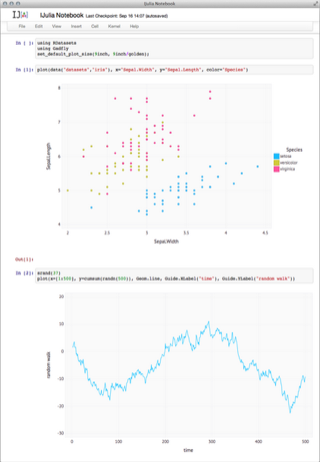# Fun with Julia, metaprogramming and Sublime Text

September 29, 2013, [MD]Julia is a newish programming language developed at MIT, targetting data analysis and scientific computing. You can read the creators of the language describe why they created Julia, or see an example of linear regressions. I've been spending a lot of time programming R in RStudio, which is a great IDE for R (although it often crashes, unfortunately), and really come to appreciate the power of the programming ecosystem. However, as many will agree (see the R Inferno), the underlying language that R is built on leaves much to desire, it's got a lot of quirks, a weird object orientation system "bolted on", and is also slow (overcome by many packages including C extensions, but that makes hacking on it much harder).One obvious contender is Python with NumPy and SciPy, and the amazing IPython Notebook (great video) and Pandas. There is clearly a lot of momentum in this community, with books being published, tutorials etc. I also see interesting uses of embedded Python for scripting on for example Quantopian.

Another is Julia, which I mentioned above. It's very early days, the language is developing so quickly that you are encouraged to compile from git, and update frequently, but it seems to have a very solid foundation, and an incredibly welcoming community. Although I really don't have time these days, I decided to sit down and play with it a bit -- I especially wanted to look at some of the large tables I'm working on in the context of MOOC research, and see how functional their DataFrames are.

Julia is not an object-oriented language, which I'm used to, but a functional language (I got a bit of an introduction to FP languages after playing around with Clojure), and it uses types and multiple dispatch to select which function gets run. In an object-oriented language, the reason why both `2 + 2` and `"hello" + "peter"` work, is that `2` and `"hello"` are objects, and which have related methods. When Ruby parses `2+2`, it actually transforms it to `2.+(2)`, ie. calling the method `+` on the class Integer. In Julia (the following is as much as I understand, I'm still learning) functions specify which arguments they accept, their type signatures. For example, the function for adding to integers is below:

``````+(x::Int64,   y::Int64)   = box(Int64,add_int(unbox(Int64,x),unbox(Int64,y)))
``````

It declares that the `+` function takes two Ints, and then specifies what is done with them. However, if you add two DataFrames, it goes to this function:

``````function (\$f)(a::DataFrame, b::DataFrame)
``````

It's `\$f`, because the DataFrames package somehow programmatically generates matching functions, this function is found within this loop:

``````for f in array_arithmetic_operators
@eval begin
``````

These functions can be spread over different files, and even packages (the DataFrames `+` is only available if the DataFrames package is loaded). Luckily, Julia has some great support for introspection. For example, you can see all the function definitions supported for `+`:

``````julia> methods(+)
# 118 methods for generic function "+":
+(x::Bool,y::Bool) at bool.jl:38
+(x::Int64,y::Int64) at int.jl:36
+(x::Union(SubArray{Bool,N,A<:Array{T,N},I<:(Union(Range{Int64},Int64,Range1{Int64})...,)},Array{Bool,N}),y::Union(SubArray{Bool,N,A<:Array{T,N},I<:(Union(Range{Int64},Int64,Range1{Int64})...,)},Array{Bool,N})) at array.jl:973
+{S,T}(A::Union(SubArray{S,N,A<:Array{T,N},I<:(Union(Range{Int64},Int64,Range1{Int64})...,)},Array{S,N}),B::Union(SubArray{T,N,A<:Array{T,N},I<:(Union(Range{Int64},Int64,Range1{Int64})...,)},Array{T,N})) at array.jl:917
...
``````

I'm just showing the top of a long listing. You can see that it also tracks where each definition is defined (file path and line number). It also has a great macro called `@which`, which shows you exactly which function will be called, given a specific expression. (My understanding is that it has to be a macro, because if it were a normal function, the contents would first be evaluated before the function were called.)

Here's an example:

``````julia> @which 2+2
+(x::Int64,y::Int64) at int.jl:36
``````

Here it shows us that given `2+2`, Julia will call the `+` function found in int.jl (which we know is in julia/base/), line 36. Looking at this, it struck me that it's possible to call Sublime Text from the command line using the exact same syntax, file name:line number. I wondered if it would be possible to hack this macro to automatically load up the right file in Sublime Text, focusing it on the relevant line...

So I opened up the macro `@which` (of course `@which @which 2+2` tells us where the macro is defined), and found that it calls a function called `which`, which calls a function called `whicht`, as below:

``````function whicht(f, types)
for m in methods(f, types)
lsd = m.func.code::LambdaStaticData
d = f.env.defs
while !is(d,())
if is(d.func.code, lsd)
print(STDOUT, f.env.name)
show(STDOUT, d); println(STDOUT)
return
end
d = d.next
end
end
end
``````

I am a complete beginner in Julia, and don't even properly understand the basics. To add to the difficulty, because macros are passing expressions around, they are a little bit hard to get your head around. In the end, the easiest thing was to modify the function `whicht` above, and create a new one, `whichst`:

``````function whichst(f, types)
for m in methods(f, types)
lsd = m.func.code::LambdaStaticData
file = string(lsd.file)
if !ismatch(r"\/", file)
file = string("/Users/Stian/src/julia/base/", file)
print("hi")
end
run(`subl \$(file):\$(lsd.line)`)
end
end
``````

And create a new macro `whichs` to call that function. A lot of copy+pasted code, not very DRY, hard-coded paths etc... but it worked! A simple hack, but a lot of fun -- and it will be incredibly useful as I continue my explorations of Julia. (One of the great things with the language is that most of it is written in Julia, which makes it much easier to hack on, and to understand).

Below is a quick screencast showing the use of these macros in practice:

I'm looking forward to playing more with Julia, and using this macro to help me understand the code better. And once I learn more, I might be able to rewrite it to be significantly more compact. For now, my very ugly hack is available as a gist.